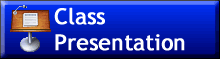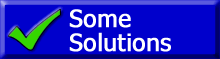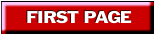This text is meant to accompany class discussions. It is not everything there is to know about energy. It is meant as a  prep for class. More detailed notes and examples are given in the class notes, presentations, and demonstrations linked below under unit resources.
Table of contents
 Page Description 0 Objectives (AP Physics 2 1 Introduction 2 Light is a particle or a wave? 2 Reflection 3 Waves 3 Speed of light 3 The wave equation 3 Electromagnetic Spectrum 3 Wave types (Transverse and Longitudinal) 3 Polorization of Waves 3 Wave Energy 3 Electromagnetic Spectrum 3 Principle of Super Position 4 Index of refraction 4 Light properties as it travels between two different mediums. 4 Snell's Law 4 Critical Angle
Units ResourcesTBATBATBATBAObjectives
Students will be able to:
1. For all equations, write what each variable stands for and its S.I. unit.
2. Identify the correct S.I. units for ALL forms of Energy.
3. Recall the name of the person who the energy unit is named after.
4. Write the equation for kinetic energy.
5. Define kinetic energy.
6. Identify when an object possesses kinetic energy.
7. Calculate the kinetic energy of an object.
8. Write the equation for potential energy due to gravity.
9. Define potential energy.
10. Identify when an object possesses potential energy.
11. Calculate the potential energy of an object.
12. Solve problems based on conservation of mechanical energy. -Neglect friction and normal forces.
13. Correctly identify the S.I. unit of “work.”
14. Define what “work” is.
15. Write the equation for “work.”
16. Calculate “work” when the force and displacement are parallel.
17. Calculate “work” when the force and displacement are not parallel.
18. Identify “work” as being positive or negative.
19. Identify “work” as being done “on” or “by” the system.
20. Calculate the work on a graph of force vs. displacement.
21. Solve problems using energy – work relationships and ratios.
22. Solve problems involving up to all two types of energies and work.
23. Recall the person who the unit of power is named after.
24. Write the two equations for power.
25. Define power 2 ways –in terms of work and in terms of force.
26. Calculate the power delivered or received by an object

Introductionby Tony Wayne ...(If you are a teacher, please feel free to use these resources in your teaching.)